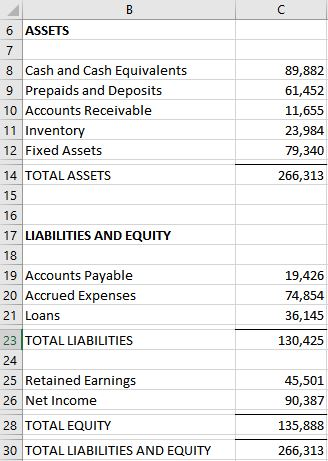## Remove Duplication in Formulas with LET

Have you ever written a formula that repeats the same expression multiple times? I know I have. One simple example is IF((A1-B1)=0,0,A1-B1). Notice how the expression A1-B1 is used twice? This is a simple example of duplicating an expression within a formula. In general, we want to try to remove/minimize such duplication to make the formula cleaner and easier to manage. Historically, defined names have been able to help with this. Now, we have a new option … LET.

## Overview

In March 2020, Microsoft announced the LET function on their Excel blog (thanks Mynda for the heads-up!). So, depending on when you are reading this and which version of Excel you have, you may or may not have access to the LET function right now.

It enables us to remove duplicate expressions from formulas. How? By letting us create names with a formula scope. If you are already familiar with defined names, you know that they have worksheet or workbook scope. Now, the LET function gives us the ability to define names with a formula scope, meaning, they are defined and recognized within a specific formula. This is very handy for eliminating duplication within formulas.

The syntax of the function:

`=LET(name1, value1, [name2], [value2], ..., calculation)`

Where:

• Name/value arguments come in pairs
• The name arguments are the formula-scoped names
• The value arguments are the expressions/references
• The calculation argument returns the result to the cell

Let’s see it in action with an illustration.

## Illustration

Let’s say we have a some type of financial report. Perhaps it is a balance sheet and looks a bit like this:I know that Total Assets must equal Total Liabilities and Equity. If the difference between them is zero, the report is OK. Otherwise, it has a problem that I need to fix before I distribute it. So, I want to check to confirm that my report looks good.

So, I write the following formula to test this:

`=IF((C14-C30)=0, "OK", "Off by:"&C14-C30)`

This formula computes the difference (C14-C30) and compares it to zero. If the difference is equal to zero, the report is OK. Otherwise, the report is Off by the amount of the difference.

Notice that the difference C14-C30 is computed twice. This means we have duplicated the expression C14-C30 … and in general we want to eliminate/reduce such duplication.

The idea behind the LET function is to define and compute an expression once and then reference it within the formula as needed.

If we wanted to replace the formula using LET, our first step would be to define the difference expression and name it, like this:

`=LET(diff,C14-C30, ...`

Here, we assign the name diff to the expression C14-C30. This name, diff, can be used as desired in the formula. So, we could finish it out like this:

`=LET(diff,C14-C30, IF(diff=0,"OK","Off by:"&diff))`

So, Jeff … big deal … this actually seems more complicated that the original formula. Well, here’s the thing. The LET version is easier to update and maintain over time. Let’s say we wanted to wrap a ROUND function around the expression, or change a cell reference. In the first version of the formula, we would have to make that change multiple times (and hopefully we realize that we need to make that change multiple times.)

In the LET version, we change it once. This makes the formula more reliable and easier to maintain over time.

This was a simple example, but as you can imagine, the usefulness of this function increases with the complexity of our formulas. The key is that it helps us reduce/minimize duplication within formulas. This gives us a performance boost and makes the formula easier to maintain going forward.

So, as you are writing formulas, if you catch yourself referencing the same expression multiple times, consider using LET to eliminate the duplication!

## Sample file

Posted in ,### Jeff Lenning

I love sharing the things I've learned about Excel, and I built Excel University to help me do that. My motto is: Learn Excel. Work Faster.

### Want to learn Excel?

Our Campus Pass includes access to our entire Undergrad and Masters catalog. Gamification ensures it is the most fun you can have learning Excel :)

1.Excel easy on April 28, 2020 at 7:42 pm

A detailed article, quite good. Thank you for sharing.

2.Christian T Stokes on April 29, 2020 at 7:47 am

Can you have multiple LETs in one formula?

3.Barbara on April 29, 2020 at 10:59 am

Love this new LET Function! Thanks for sharing!!! I use duplication all the time so it would be nice to be able to use this in my desktop app, unfortunately, my O365 Excel has LET, but my desktop Excel 2016 does not.

4.Donna on April 29, 2020 at 11:28 am

Awesome! I have some nested calc’s that use the same large calc a number of times, this is awesome!

5.Cynthia on May 6, 2020 at 11:04 am

When working with multiple versions of Excel, if you save a book as a template will it allow those with non-O365 to be able to use the book?

!
!